﻿ 基于EGARCH模型下的沪深300指数风险研究 Risk Research of Shanghai and Shenzhen 300 Index Based on EGARCH Model

Finance
Vol. 09  No. 04 ( 2019 ), Article ID: 31259 , 9 pages
10.12677/FIN.2019.94042

Risk Research of Shanghai and Shenzhen 300 Index Based on EGARCH Model

Xiaoxiao Zhang, Kebo Lv

School of Mathematical Sciences, Ocean University of China, Qingdao Shandong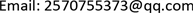Received: Jun. 21st, 2019; accepted: Jul. 4th, 2019; published: Jul. 15th, 2019ABSTRACT

In this paper, based on the assumption that the Shanghai and Shenzhen 300 Index is based on positive distribution, student t distribution, biased student t distribution, and GED distribution, the EGARCH model is used to model the leverage effect of yield volatility, and the VaR model is used for backtesting. It is shown that the volatility model based on the GED distribution is significantly better than the EGARCH model under other distributions.

Keywords:Shanghai and Shenzhen 300 Stock Index, Asymmetry, EGARCH Model1. 引言

Bollerslev (1986)  提出了广义的ARCH模型即GARCH模型，是在Engle  的ARCH模型基础上对方差的表现形式进行了直接的线性扩展，GARCH模型应用更为广泛，解决了高阶ARCH模型待估参数过多导致模型不够精确的问题。建立基于正态分布、学生t分布、有偏学生t分布、和广义误差分布下指数EGARCH模型研究沪深300指数收益率序列的特点  - ，对模型进行回溯检验，建立VaR风险度量模型对所拟合的一系列GARCH类模型进行评估，选择拟合效果较好的模型对波动率进行预测。

2. 模型设定

2.1. EGARCH模型

$\left\{\begin{array}{l}{r}_{t}={\mu }_{t}+{\alpha }_{t}\\ {\alpha }_{t}={\sigma }_{t}{\epsilon }_{t}\\ \mathrm{ln}\left({\sigma }_{t}^{2}\right)=\omega +\sum _{j=1}^{m}{\beta }_{j}\mathrm{ln}\left({\sigma }_{t-j}^{2}\right)+\sum _{i=1}^{n}{\alpha }_{i}\ast \left(|\frac{{\epsilon }_{t-1}}{{\sigma }_{t-1}}|+{\gamma }_{i}\frac{{\epsilon }_{t-1}}{{\sigma }_{t-1}}\right)\end{array}$

2.2. VaR模型基础理论

VaR关注资产损益的尾部特征，提高VaR计算的准确性。VAR通俗讲“有的把握在今后的N天内损失 $\Delta p$ 不会大于VaR”，公式表达为 $\text{prob}\left(\Delta p\le \text{VaR}\right)=\alpha$ 。VaR是评价金融风险的度量，其定义为金融头寸在持有期内以某个概率发生的最大损失，所以损失函数的正负取决于金融头寸，空头的损失就是资产收益率，多头的损失为其相反数，VaR的计算公式为持有期内的 $\text{VaR}={Z}_{\alpha }{\sigma }_{t}\sqrt{\Delta t}$ ( $\Delta t$ 为持有期)，其中 ${Z}_{\alpha }$ 为置信度  对应的某种分布的分位数，收益率序列计算的标准差作为收益率的波动率。

$\text{LR}=-2\mathrm{ln}\left[{\left(1-P\right)}^{N-n}{P}^{n}\right]+2\mathrm{ln}\left[{\left(1-\frac{n}{N}\right)}^{N-n}{\left(\frac{n}{N}\right)}^{n}\right]$

3. 实证研究

3.1. 数据选取

3.2. 数据检验过程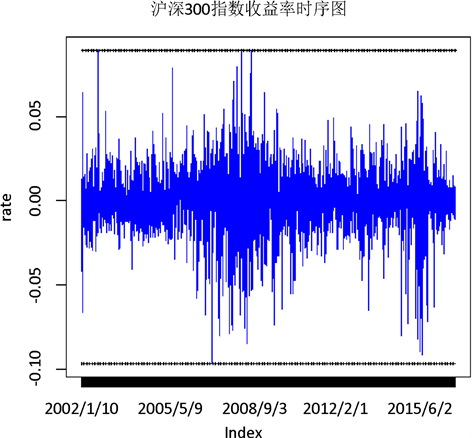Figure 1. Logarithmic yield sequence chart of csi 300 index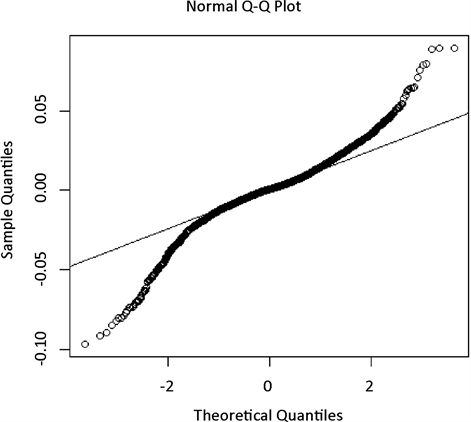Figure 2. Log return sequence QQ diagram of csi 300 indexTable 1. Table of basic statistical characteristics

3.3. 序列平稳性检验

3.4. 拟合ARMA模型

ARMA(p,q)模型方程为

${r}_{t}={\beta }_{0}+{\beta }_{1}{r}_{t-1}+{\beta }_{2}{r}_{t-2}+\cdots +{\beta }_{p}{r}_{t-p}+{\epsilon }_{t}+{\alpha }_{1}{\epsilon }_{t-1}+\cdots +{\alpha }_{q}{\epsilon }_{t-q}$

ARMA模型中p、q定阶一般根据自相关、偏自相关图系数拖尾与截尾的情况来确定阶数。本文收益率序列的自相关、偏自相关图不易观察确定ARMA(p,q)模型中的阶数，也为了避免个人经验的不足导致模型识别不准确，笔者在R软件中采用模型信息量越小模型越优的准则对模型进行自动定阶，最终确立p与q均为1。

${r}_{t}=0.000206-0.765201{r}_{t-1}+{\epsilon }_{t}-0.78461{\epsilon }_{t-1}$

p值(0.156971) 0.0000 0.0000

3.5. ARCH效应检验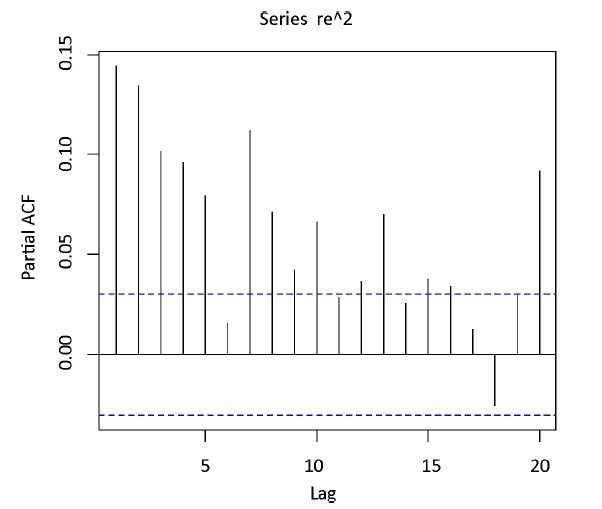Figure 3. Partial autocorrelation of residual sequence squared

3.6. 建立EGARCH模型Table 3. Estimation results of index GARCH parameters

$\mathrm{log}{\sigma }_{t}^{2}=-0.0697+0.1393\frac{{\epsilon }_{t-1}}{{\sigma }_{t-1}}-0.0137|\frac{{\epsilon }_{t-1}}{{\sigma }_{t-1}}|+0.9918+\mathrm{log}{\sigma }_{t-1}^{2}$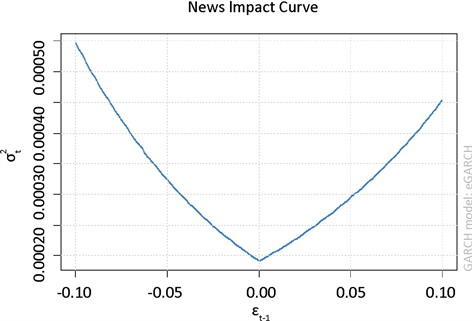Figure 4. Curve of information impact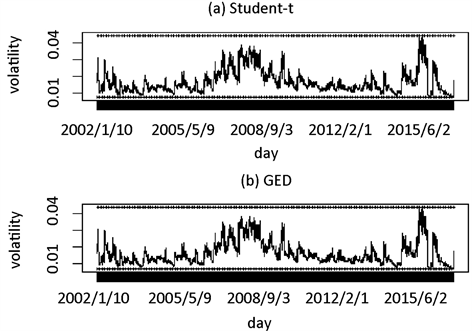Figure 5. Volatility under t distribution and GED distribution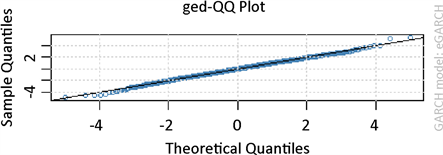Figure 6. QQ diagram of residual error

3.7. VaR回溯测试

LR统计量越小说明模型预测效果越好，在正态分布95%的置信水平下，除多头有偏学生t分布、空头正态分布没有通过检验外均通过了检验，即不拒绝模型正确的原假设。在t分布下的LR值相较于正态

3.8. 预测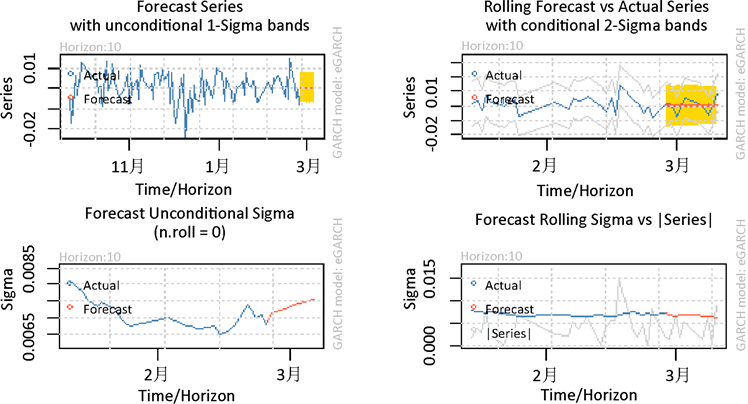Figure 7. Forecast chart of return rate and volatility of csi 300 index

4. 结论及政策建议

Risk Research of Shanghai and Shenzhen 300 Index Based on EGARCH Model[J]. 金融, 2019, 09(04): 341-349. https://doi.org/10.12677/FIN.2019.94042

1. 1. Bollerslev, T. (1986) Generalized Autoregressive Conditional Heteroskedasticity. Journal of Econometrics, 31, 307-327. https://doi.org/10.1016/0304-4076(86)90063-1

2. 2. Engle, R.F. (1982) Autoregressive Conditional He-teroscedasticity with Estimates of the Variance of United Kingdom Inflation. Econometrica: Journal of the Econometric Society, 50, 987-1007. https://doi.org/10.2307/1912773

3. 3. Nelson, D.B. (1991) Conditional Heteroskedasticity in Asset Returns: A New Approach. Econometrica: Journal of the Econometric Society, 59, 347-370. https://doi.org/10.2307/2938260

4. 4. Engle, R.F. and Ng, V.K. (1993) Measuring and Testing the Impact of News on Volatility. The Journal of Finance, 48, 1749-1778. https://doi.org/10.1111/j.1540-6261.1993.tb05127.x

5. 5. Duan, J.C. (1995) The GARCH Option Pricing Model. Mathematical Finance, 5, 13-32. https://doi.org/10.1111/j.1467-9965.1995.tb00099.x

6. 6. Giambastiani, B.M.S. (2007) Evoluzione Idrologica ed Idrogeologica della Pineta di San Vitale (Ravenna). PhD Thesis, Bologna University, Bologna.

7. 7. Liu, X., Cheng, S., Wang, S., et al. (2008) An Empirical Study on Information Spillover Effects between the Chinese Copper Futures Market and Spot Market. Physica A: Statistical Mechanics and Its Applications, 387, 899-914. https://doi.org/10.1016/j.physa.2007.09.044

8. 8. Kupiec, P. (1995) Techniques for Verifying the Accuracy of Risk Measurement Models. The Journal of Derivatives, 3, 73-84. https://doi.org/10.3905/jod.1995.407942

9. 9. 赖文炜, 陈云. 我国股指期货市场波动的非对称性及其国际比较研究[J]. 商业研究, 2015, 57(5): 73-78.

10. 10. 姚京, 李仲飞. VaR估计中的模型风险——检验方法与实证研究[J]. 管理评论, 2005, 17(10): 3-7+54-63.

11. 11. 蒋虹, 曲丹丹. 基于VaR的沪深300股指期货风险管理实证研究[J]. 经济问题, 2008(12): 119-122.

12. 12. 王美今, 王华. 基于GARCH-t的上海股票市场险值分析[J]. 数量经济技术经济研究, 2002, 19(3): 106-109.

13. 13. 曹原. 基于t-GARCH模型的风险价值VaR及其在我国股票市场中的实证分析[J]. 理论研究, 2012(2): 108-109